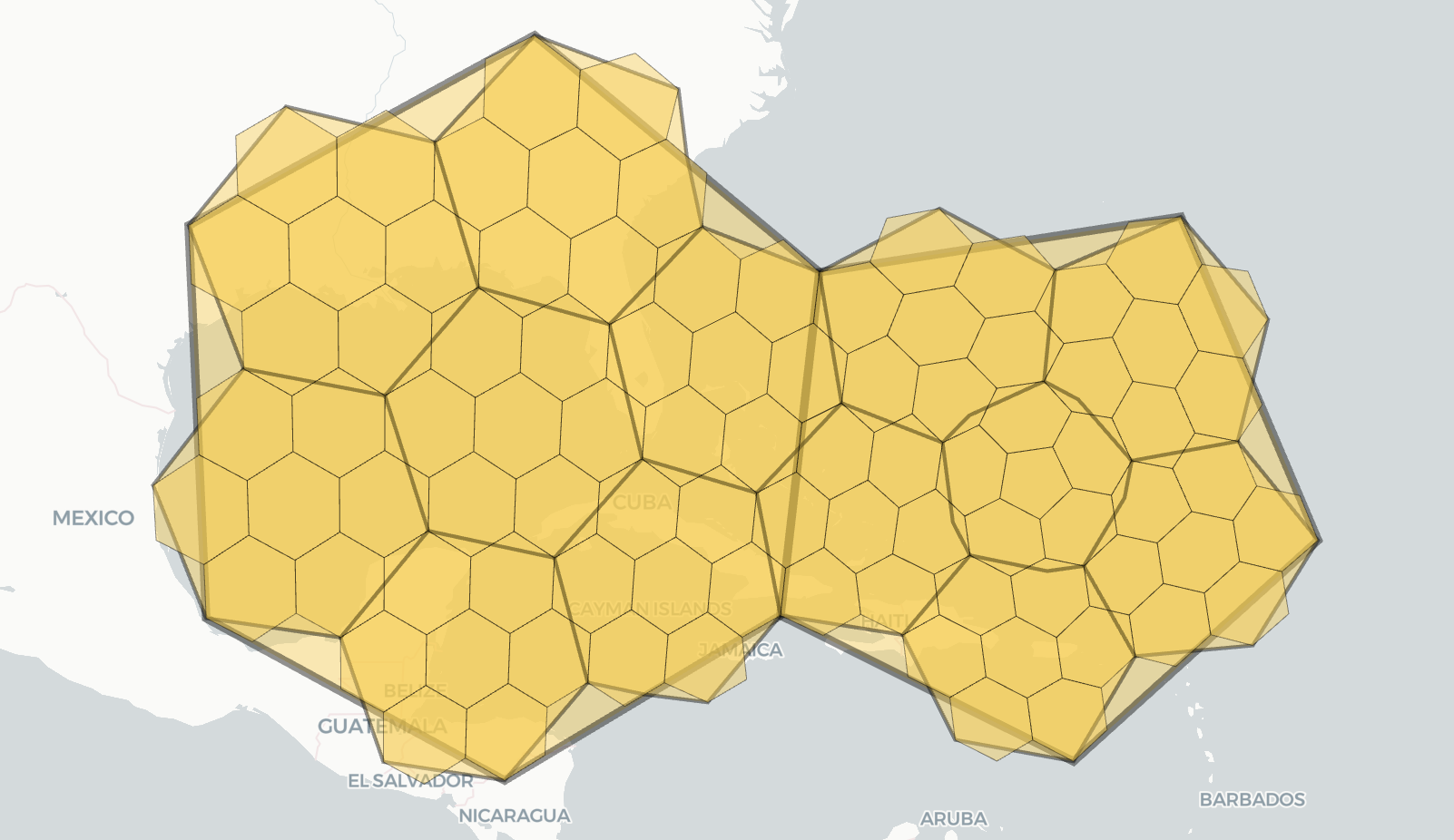Version: 4.x

# Tables of Cell Statistics Across Resolutions

## Cell counts​

We list the number of hexagons and pentagons at each H3 resolution. There are always exactly $12$ pentagons at every resolution.

ResTotal number of cellsNumber of hexagonsNumber of pentagons
012211012
184283012
25,8825,87012
341,16241,15012
4288,122288,11012
52,016,8422,016,83012
614,117,88214,117,87012
798,825,16298,825,15012
8691,776,122691,776,11012
94,842,432,8424,842,432,83012
1033,897,029,88233,897,029,87012
11237,279,209,162237,279,209,15012
121,660,954,464,1221,660,954,464,11012
1311,626,681,248,84211,626,681,248,83012
1481,386,768,741,88281,386,768,741,87012
15569,707,381,193,162569,707,381,193,15012

## Cell areas​

caution

Cell areas are computed with a spherical model of the earth using the authalic radius given by WGS84/EPSG:4326.

### Average area in km2​

The area of an H3 cell varies based on its position relative to the icosahedron vertices. We show the average hexagon areas for each resolution. All pentagons within a resolution have the same area.

ResAverage Hexagon Area (km2)Pentagon Area* (km2)Ratio (P/H)
04,357,449.4160783812,562,182.1629554960.5880
1609,788.441794133328,434.5862464690.5386
286,801.78039899744,930.8984978790.5176
312,393.4346550886,315.4722675160.5096
41,770.347654491896.5823831410.5064
5252.903858182127.7855830230.5053
636.12906216418.2387495480.5048
75.1612933602.6046693970.5047
80.7373275980.3720480380.5046
90.1053325130.0531471950.5046
100.0150475020.0075923180.5046
110.0021496430.0010846090.5046
120.0003070920.0001549440.5046
130.0000438700.0000221350.5046
140.0000062670.0000031620.5046
150.0000008950.0000004520.5046

*: Within a given resolution, all pentagons have the same area.

### Average area in m2​

Here are the same areas, but in m2.

ResAverage Hexagon Area (m2)Pentagon Area* (m2)
04,357,449,416,078.3922,562,182,162,955.496
1609,788,441,794.134328,434,586,246.469
286,801,780,398.99744,930,898,497.879
312,393,434,655.0886,315,472,267.516
41,770,347,654.491896,582,383.141
5252,903,858.182127,785,583.023
636,129,062.16418,238,749.548
75,161,293.3602,604,669.397
8737,327.598372,048.038
9105,332.51353,147.195
1015,047.5027,592.318
112,149.6431,084.609
12307.092154.944
1343.87022.135
146.2673.162
150.8950.452

*: Within a given resolution, all pentagons have the same area.

### Hexagon min and max areas​

The area of an H3 cell varies based on its position relative to the icosahedron vertices. We compute the minimum and maximum values for the hexagon areas (excluding the pentagons) at each resolution, and show their ratio.

ResMin Hexagon Area (km^2)Max Hexagon Area (km^2)Ratio (max/min)
04,106,166.3344639154,977,807.0274420121.212276
1447,684.201817940729,486.8752753441.629468
256,786.622889474104,599.8072189251.841980
37,725.50576963914,950.7733013791.935248
41,084.0056353632,135.9869839651.970457
5153.766244448305.1443087791.984469
621.91002101343.5921116851.989597
73.1268360306.2274459051.991613
80.4465261740.8896351571.992347
90.0637802270.1270907371.992635
100.0091109810.0181558201.992740
110.0013015420.0025936891.992782
120.0001859330.0003705271.992797
130.0000265620.0000529321.992802
140.0000037950.0000075621.992805
150.0000005420.0000010801.992805

## Edge lengths​

caution

Edge lengths are computed with a spherical model of the earth using the authalic radius given by WGS84/EPSG:4326. Average edge lengths were calculated exactly for resolutions 0 through 6 and extrapolated for finer resolutions.

ResAverage edge length (Km)
01281.256011
1483.0568391
2182.5129565
368.97922179
426.07175968
59.854090990
63.724532667
71.406475763
80.531414010
90.200786148
100.075863783
110.028663897
120.010830188
130.004092010
140.001546100
150.000584169

## Appendix: Methodology​Hexagons have 7 hexagon children. Pentagons have 6 children: 5 hexagons and 1 pentagon.

### Cell counts​

By definition, resolution 0 has $110$ hexagons and $12$ pentagons, for a total of $122$ cells.

In fact, every H3 resolution has exactly $12$ pentagons, which are always centered at the icosahedron vertices; the number of hexagons increases with each resolution.

Formula

Accounting for both hexagons and pentagons, the total number of cells at resolution $r$ is

$c(r) = 2 + 120 \cdot 7^r.$

#### Derivation of the cell count formula​

We can derive the formula above with the following steps.

First, let $h(n)$ be the number of children $n \geq 0$ resolution levels below any single hexagaon. Any hexagon has $7$ immediate children, so recursion gives us that

$h(n) = 7^n.$

Next, let $p(n)$ be the number of children $n \geq 0$ resolution levels below any single pentagon. Any pentagon has $5$ hexagonal immediate children and $1$ pentagonal immediate child. Thus, $p(0) = 1$ and $p(1) = 6$.

For $n \geq 1$, we get the general recurrence relation

\begin{aligned} p(n) &= 5 \cdot h(n-1) + p(n-1) \\ &= 5 \cdot 7^{n-1} + p(n-1). \end{aligned}

For $n \geq 0$, after working through the recurrence, we get that

\begin{aligned} p(n) &= 1 + 5 \cdot \sum_{k=1}^n\ 7^{k-1} \\ &= 1 + 5 \cdot \frac{7^n - 1}{6}, \end{aligned}

using the closed form for a geometric series.

Finally, using the closed forms for $h(n)$ and $p(n)$, and the fact that (by definition) resolution 0 has $12$ pentagons and $110$ hexagons, we get the closed form for the total number of cells at resolution $r$ as

\begin{aligned} c(r) &= 12 \cdot p(r) + 110 \cdot h(r) \\ &= 2 + 120 \cdot 7^r. \end{aligned}

#### Jupyter notebook​

A notebook to produce the cell count table above can be found here.

### Cell areas​

Cell areas are computed with a spherical model of the earth using the authalic radius given by WGS84/EPSG:4326.

The h3-py-notebooks repo has notebooks for producing the average cell area table and the min/max area table.

todo